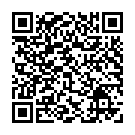## Y5 Number - Multiplication and Division - Interactive Quiz

### Related Worksheets

An interactive quiz which test your understanding of all the Multiplication and Division objectives in the Year 5 curriculum. Choose one objective or multiple objectives. You can save, or print, your test results as a pdf at the end of the quiz. Ideal for formative or summative assessment.

Alternatively, you can use the Interactive Maths Quiz which includes objectives from all strands of the Year 5 curriculum.

This quiz tests the following objectives:

• identify multiples and factors, including finding all factor pairs of a number, and common factors of 2 numbers
• know and use the vocabulary of prime numbers, prime factors and composite (non-prime) numbers
• establish whether a number up to 100 is prime and recall prime numbers up to 19
• multiply numbers up to 4 digits by a one- or two-digit number using a formal written method, including long multiplication for two-digit numbers
• multiply and divide numbers mentally, drawing upon known facts
• divide numbers up to 4 digits by a one-digit number using the formal written method of short division and interpret remainders appropriately for the context
• multiply and divide whole numbers and those involving decimals by 10, 100 and 1,000
• recognise and use square numbers and cube numbers, and the notation for squared (²) and cubed (³)
• solve problems involving multiplication and division, including using their knowledge of factors and multiples, squares and cubes
• solve problems involving addition, subtraction, multiplication and division and a combination of these, including understanding the meaning of the equals sign
• solve problems involving multiplication and division, including scaling by simple fractions and problems involving simple ratesScan to open this game on a mobile device. Right-click to copy and paste it onto a homework sheet.